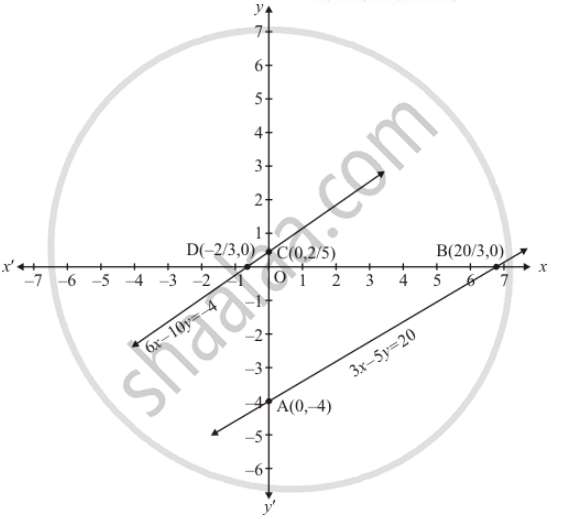Share

# Show Graphically that Each One of the Following Systems of Equations is Inconsistent (I.E. Has No Solution) : 3x − 5y = 20 6x − 10y = −40 - CBSE Class 10 - Mathematics

ConceptGraphical Method of Solution of a Pair of Linear Equations

#### Question

Show graphically that each one of the following systems of equations is inconsistent (i.e. has no solution) :

3x − 5y = 20

6x − 10y = −40

#### Solution

The given equations are

3x - 5y = 20 ...(i)

6x - 10y = -4 ...(ii)

Putting x = 0 in equation (i)  we get

=> 3 xx 0 - 5y = 20

=> y = -4

x= 0, y = -4

Putting y = 0 in equation (i) we get

=> 3x - 5 xx 0 = 20

=> x = 20/3

x= 20/3, y = 0

Use the following table to draw the graph.

 x 0 20/3 y -4 0

Draw the graph by plotting the two points  A(0,-4), B(20/3, 0) from tableGraph of the equation ...(ii)

6x - 10y = -4 ....(ii)

Putting x = 0 in equaion (ii) we get

=> 6 xx  0 - 10y = -4

=> y = 2/5

x = 2/5, y = 0

Putting y = 0 in equation (ii) we get

=> 6x - 10 xx 0 = -4

=> x = -2/3

x = -2/3, y = 0

Use the following table to draw the graph.

 x 0 -2/3 y 2/5 0

Draw the graph by plotting the two points C(0, -4), D(20/3, 0) from table.

Here we see that the two lines are parallel

Hence the given system of equations has no solution.

Is there an error in this question or solution?

#### APPEARS IN

Solution Show Graphically that Each One of the Following Systems of Equations is Inconsistent (I.E. Has No Solution) : 3x − 5y = 20 6x − 10y = −40 Concept: Graphical Method of Solution of a Pair of Linear Equations.
S